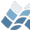NV5 GEOSPATIAL SOFTWARECMAPPLY

CMAPPLY

## Author

Craig B. Markwardt, NASA/GSFC Code 662, Greenbelt, MD 20770
craigm@lheamail.gsfc.nasa.gov

## Purpose

Applies a function to specified dimensions of an array

Arrays

## Calling Sequence

XX = CMAPPLY(OP, ARRAY, DIMS, [/DOUBLE], [TYPE=TYPE])

## Description

CMAPPLY will apply one of a few select functions to specified
dimensions of an array. Unlike some IDL functions, you *do* have
a choice of which dimensions that are to be "collapsed" by this
function. Iterative loops are avoided where possible, for
performance reasons.
The possible functions are: (and number of loop iterations:)
+ - Performs a sum (as in TOTAL) number of collapsed dimensions
AND - Finds LOGICAL "AND" (not bitwise) same
OR - Finds LOGICAL "OR" (not bitwise) same
* - Performs a product LOG_2[no. of collapsed elts.]
MIN - Finds the minimum value number of collapsed dimensions
MAX - Finds the maximum value same
MEDIAN- Finds the median value same
USER - Applies user-defined function no. of output elements
It is possible to perform user-defined operations arrays using
CMAPPLY. The OP parameter is set to 'USER:FUNCTNAME', where
FUNCTNAME is the name of a user-defined function. The user
defined function should be defined such that it accepts a single
parameter, a vector, and returns a single scalar value. Here is a
prototype for the function definition:
FUNCTION FUNCTNAME, x, KEYWORD1=key1, ...
scalar = ... function of x or keywords ...
RETURN, scalar
END
The function may accept keywords. Keyword values are passed in to
CMAPPLY through the FUNCTARGS keywords parameter, and passed to
the user function via the _EXTRA mechanism. Thus, while the
definition of the user function is highly constrained in the
number of positional parameters, there is absolute freedom in
passing keyword parameters.
It's worth noting however, that the implementation of user-defined
functions is not particularly optimized for speed. Users are
encouraged to implement their own array if the number of output
elements is large.

## Inputs

OP - The operation to perform, as a string. May be upper or lower
case.
If a user-defined operation is to be passed, then OP is of
the form, 'USER:FUNCTNAME', where FUNCTNAME is the name of
the user-defined function.
ARRAY - An array of values to be operated on. Must not be of type
STRING (7) or STRUCTURE (8).

## Optional Inputs

DIMS - An array of dimensions that are to be "collapsed", where
the the first dimension starts with 1 (ie, same convention
as IDL function TOTAL). Whereas TOTAL only allows one
dimension to be added, you can specify multiple dimensions
to CMAPPLY. Order does not matter, since all operations
are associative and transitive. NOTE: the dimensions refer
to the *input* array, not the output array. IDL allows a
maximum of 8 dimensions.
DEFAULT: 1 (ie, first dimension)

## Keywords

DOUBLE - Set this if you wish the internal computations to be done
in double precision if necessary. If ARRAY is double
precision (real or complex) then DOUBLE=1 is implied.
DEFAULT: not set
TYPE - Set this to the IDL code of the desired output type (refer
to documentation of SIZE()). Internal results will be
rounded to the nearest integer if the output type is an
integer type.
DEFAULT: same is input type
FUNCTARGS - If OP is 'USER:...', then the contents of this keyword
are passed to the user function using the _EXTRA
mechanism. This way you can pass additional data to
your user-supplied function, via keywords, without
using common blocks.
DEFAULT: undefined (i.e., no keywords passed by _EXTRA)

## Return Value

An array of the required TYPE, whose elements are the result of
the requested operation. Depending on the operation and number of
elements in the input array, the result may be vulnerable to
overflow or underflow.

## Examples

Shows how CMAPPLY can be used to total the second dimension of the
array called IN. This is equivalent to OUT = TOTAL(IN, 2)
IDL> IN = INDGEN(5,5)
IDL> OUT = CMAPPLY('+', IN, )
IDL> HELP, OUT
OUT INT = Array
Second example. Input is assumed to be an 5x100 array of 1's and
0's indicating the status of 5 detectors at 100 points in time.
The desired output is an array of 100 values, indicating whether
all 5 detectors are on (=1) at one time. Use the logical AND
operation.
IDL> IN = detector_status ; 5x100 array
IDL> OUT = CMAPPLY('AND', IN, ) ; collapses 1st dimension
IDL> HELP, OUT
OUT BYTE = Array
(note that MIN could also have been used in this particular case,
although there would have been more loop iterations).
Third example. Shows sum over first and third dimensions in an
array with dimensions 4x4x4:
IDL> IN = INDGEN(4,4,4)
IDL> OUT = CMAPPLY('+', IN, [1,3])
IDL> PRINT, OUT
408 472 536 600
Fourth example. A user-function (MEDIAN) is used:
IDL> IN = RANDOMN(SEED,10,10,5)
IDL> OUT = CMAPPLY('USER:MEDIAN', IN, 3)
IDL> HELP, OUT
OUT FLOAT = Array[10, 10]
(OUT(i,j) is the median value of IN(i,j,*))

## Modification History

Mar 1998, Written, CM
Changed usage message to not bomb, 24 Mar 2000, CM
Signficant rewrite for *, MIN and MAX (inspired by Todd Clements
<Todd_Clements@alumni.hmc.edu>); FOR loop indices are now type
LONG; copying terms are liberalized, CM, 22, Aug 2000
More efficient MAX/MIN (inspired by Alex Schuster), CM, 25 Jan
2002
Make new MAX/MIN actually work with 3d arrays, CM, 08 Feb 2002
Add user-defined functions, ON_ERROR, CM, 09 Feb 2002
Correct bug in MAX/MIN initialization of RESULT, CM, 05 Dec 2002
Correct bug in CMAPPLY_PRODUCT implementation when there are an
odd number of values to combine, CM 26 Jul 2006
Add fast IDL versions of '*', 'MEDIAN', 'MIN' and 'MAX', where
IDL supports it, CM 26 Jul 2006

© 2023 NV5 Geospatial |  Legal